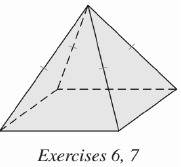Chapter 9.CT, Problem 7CT### Elementary Geometry for College St...

6th Edition
Daniel C. Alexander + 1 other
ISBN: 9781285195698

#### Solutions

Chapter
Section### Elementary Geometry for College St...

6th Edition
Daniel C. Alexander + 1 other
ISBN: 9781285195698
Textbook Problem
1 views

# Find the volume of the regular square pyramid shown if each edge of the base measures 5 ft and the altitude measures 6 ft. ______________To determine

To find:

The volume of the regular square pyramid.

Explanation

Given:

For a regular square pyramid with each edge of the base measures 5 ft. and the altitude measures is 6 ft.

Formula used:

The volume of the pyramid is V=13Bh.

Calculation:

Since the base is a square with each edge measuring 5 ft, the base area is

B=55=25 ft2

### Still sussing out bartleby?

Check out a sample textbook solution.

See a sample solution

#### The Solution to Your Study Problems

Bartleby provides explanations to thousands of textbook problems written by our experts, many with advanced degrees!

Get Started

#### In Exercises 69-74, rationalize the numerator. 71. 2yx

Applied Calculus for the Managerial, Life, and Social Sciences: A Brief Approach

#### Polar coordinates of the point with rectangular coordinates (5, 5) are: (25, 0)

Study Guide for Stewart's Single Variable Calculus: Early Transcendentals, 8th

#### Self Check 5 Solve 35+7x+2=2

College Algebra (MindTap Course List)